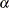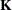LSMESTIMATE Statement

LSMESTIMATE model-effect <'label'> values <divisor=n> <, …<'label'> values <divisor=n>></ options> ;

The LSMESTIMATE statement provides a mechanism for obtaining custom hypothesis tests among least squares means.

Table 75.30 summarizes the options available in the LSMESTIMATE statement.

Table 75.30: LSMESTIMATE Statement Options

Option

Description

Construction and Computation of LS-Means

Modifies covariate values in computing LS-means

Computes separate margins

Specifies a list of values to divide the coefficients

Specifies the weighting scheme for LS-means computation as determined by a data set

Tunes estimability checking

Degrees of Freedom and p-values

Determines the method for multiple-comparison adjustment of LS-means differences

ALPHA=Determines the confidence level ()

Performs one-sided, lower-tailed inference

Adjusts multiple-comparison p-values further in a step-down fashion

TESTVALUE=

Specifies values under the null hypothesis for tests

Performs one-sided, upper-tailed inference

Statistical Output

Constructs confidence limits for means and mean differences

Displays the correlation matrix of LS-means

Displays the covariance matrix of LS-means

Prints thematrix

Prints thematrix

Produces a joint F or chi-square test for the LS-means and LS-means differences

Specifies the seed for computations that depend on random numbers

For details about the syntax of the LSMESTIMATE statement, see the section LSMESTIMATE Statement in Chapter 19: Shared Concepts and Topics.